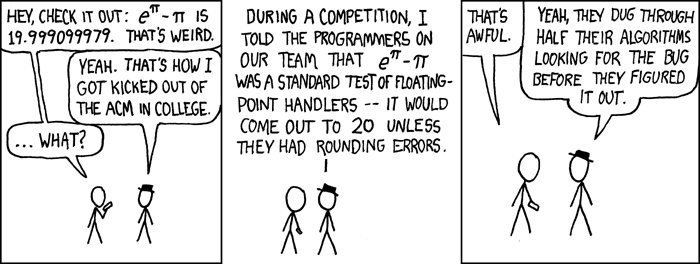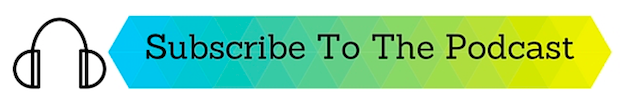From time to time we see the issue raised where floating point values are not exact like we can write in the code editor or on paper. Usually the confusion or complaint is worded like “I can’t get my double value to be precise like in the string” or “It’s not the same as I get doing it by hand”.

Unfortunately, because digital computers are based entirely on binary, they use powers of 2 and this is, by the very design of floating point values, not going to be precise on any CPU using the IEEE formats.

Let me explain: when you and I do math by hand we all have internalized the rules that decimals are stated as positional values:

1 = 100
10 = 101

And that the fraction parts are also powers of 10:

.1 = 1 / 10 = 1 / (101)
.01 = 1 / 100 = 1 / (102)
.001 = 1 / 1000 = 1 / (103)

We could write 138 as 1*(102) + 3*(101) + 8*(100)

On our computers things are similar but instead of powers of 10, they use power of 2:

1 = 20 (or as a binary literal in Xojo as &b01)
2 = 21 (or as a binary literal in Xojo as &b10)
3 = 20 + 21 (or as a binary literal in Xojo as &b11)

Floating point values are also the sum of powers of 2. They use the same powers of 2 for the whole number portion and, at their simplest, sums of powers of 2 that are increasingly small. There are some optimizations to this that CPU’s use to normalize things, but the basics are still based on this notation:

.5 = 1 / (21)
.25 = 1 / (22)
.125 = 1/ (23)

So when you want 1.5 that’s easy: (2^0) + (1 / (21)) or 1 + .5 = 1.5
Since 0.5 can be represented exactly using binary there’s no issue. But trying to represent a number like 0.3 will result in what is a common complaint:

(1/ 22) + (1 / 24) + (1/25) + (1/28) + (1/29) + (1/212) + (1/213)

This evaluates to:

.25 + .0125 + .03125 + .00390625 + .001953125 + .0002441402625 + 0.0001220703125 + 0.0000152587890625

The sum of which is:

0.29999084436406

And you can carry this on as long as you’d like and sum successive powers of 2, and while you can approximate 0.3 you never sum to exactly 0.3. There is no sum of successive powers of 2 that totals precisely to this fractional value, so it’s approximated as close as possible using those fractions.

Therein lies the problem. In a digital computer there are only so many bits used to represent a floating point values and they will be close but not precise.

There are entire books dedicated to the subject, should you want to learn more.